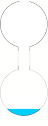Day 2, July 18 Modeling Problems Homework

Homework:

1. The following problem is a typical homework problem from a precalculus book.

Similar to our classroom explorations of the box and circle problems I would like you to first identify and explore varying relationships; then determine what varying relationships should be modeled to respond to this question.

Problem Statement: You have 40 (linear) feet of fencing with which to enclose a rectangular space for a garden.  Find the largest area that can be enclosed with this much fencing. Find the dimensions of the corresponding garden.

1. What quantities vary in this situation?
2. Identify two pairs of quantities that vary in tandem. Use the finger tool to imagine how they vary together.  Provide a brief description of what you imagined and observed by using the finger tool for each pair.
3. Using graphing calculator, construct a function that models this situation and create the graph in graphing calculator. Past your graphing calculator image into your solution.
4. Describe how the area changes as the length of one side of the garden changes from x = 0 to x = 7.
5. Determine the dimensions of the garden when the area is 45 feet.
6. What is the range of possible values that are possible for the width and length of the garden? Explain your answer in the context of the situation.2.         A tank is made from two hollow globes that are connected by a hollow stem, as shown in the figure to the right. Water is being poured into the globes from the top.

a.   Sketch a graph that shows the height of water in this configuration as a function of the water’s volume in it.

b.   A twisted, flat, rod will be placed into the configuration so that it stands at the bottom of the lower globe and ends at the top of the upper globe. A float, slotted in the middle, that cannot turn moves up the rod, so that the rod turns as the float moves upward. The rod must have a total twist of 360° as the water raises the float from the bottom to the top of the tank. Sketch a graph of “amount of turn” as a function of the water’s volume so that the rod turns at a constant rate with respect to the volume of water in the tank.